SuSiE manuscript

# Application to change point detection problem¶

Although we developed SuSiE primarily with the goal of performing variable selection in highly sparse settings -- and, in particular, for genetic fine-mapping -- the approach also has considerable potential for application to other large-scale regression problems. Here we briefly illustrate this potential by applying it to a non-parametric regression problem that at first sight seems to be ill-suited to our approach.

Specifically we focus on the "change point detection problem", which does not involve strict sparsity, and the underlying correlation structure of the explanatory variables is very different from the "blocky" covariance structure of genetic data that SuSiE was designed for. Nonetheless, we will see that SuSiE performs well here despite this (partly due to its ability to capture non-sparse signals via Bayesian Model Averaging). Consider the non-parametric regression: $$y_t = \mu_t + e_t \quad t=1,\dots,T$$ where the goal is to estimate the underlying mean, $\mu_t$, under the assumption that it varies smoothly (or, more precisely, in a spatially-structured way) with $t$. One very simple way to capture spatial structure in $\mu$ is to model it as a (sparse) linear combination of step functions: $$\mu = Xb$$ where the $j$th column of $X$ is the step function with a step at $j$ ($j = 1,\dots,(T-1)$); that is $x_{tj}=0$ for $t<=j$ and 1 for $t>j$. The $j$th element of $b$ therefore determines the change in the mean $|\mu_j-\mu_{j+1}|$, and an assumption that $b$ is sparse encapsulates an assumption that $\mu$ is spatially structured (indeed, piecewise constant). This very simple approach is essentially 0th-order trend filtering.

## SuSiE change point example¶

To illustrate the potential of SuSiE for change point estimation we applied it to a simple simulated example from the DNAcopy package.

In :
library("susieR")
library("DNAcopy")
library("changepoint")

Loading required package: zoo

Attaching package: ‘zoo’

The following objects are masked from ‘package:base’:

as.Date, as.Date.numeric

Successfully loaded changepoint package version 2.2.2
NOTE: Predefined penalty values changed in version 2.2.  Previous penalty values with a postfix 1 i.e. SIC1 are now without i.e. SIC and previous penalties without a postfix i.e. SIC are now with a postfix 0 i.e. SIC0. See NEWS and help files for further details.


The example comes from ?DNAcopy::segment (note we changed this into a single problem (single chromosome) whereas the example was originally split into two chromosomes).

In :
set.seed(51)
genomdat <- rnorm(500, sd=0.2) +
rep(c(-0.2,0.1,1,-0.5,0.2,-0.5,0.1,-0.2),c(137,87,17,49,29,52,87,42))
chrom <- rep(1,500)
maploc <- c(1:500)
test2 <- DNAcopy::segment(CNA(genomdat, chrom, maploc))

Analyzing: Sample.1

In :
pdf("cp_truth.pdf", width=8, height=5, pointsize=16)
plot(test2)
dev.off()

png: 2
In :
%preview cp_truth.pdf -s png --dpi 100

%preview cp_truth.pdf
> cp_truth.pdf (8.1 KiB):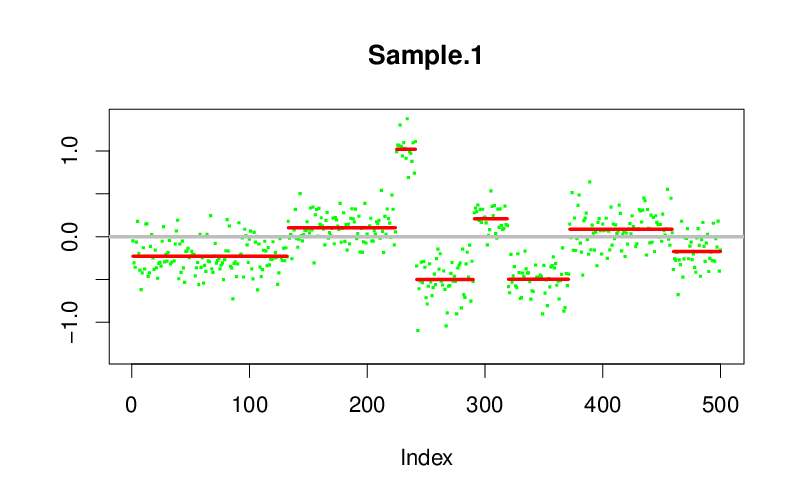We have implemented in susieR 0.6.0 a funciton susie_trendfilter which internally creates $X$ matrix with step functions in the columns to match input $y$. The algebra have been optimized to work on such trendfiltering matrices.

In :
genomdat.s = susieR::susie_trendfilter(genomdat,0)

In :
plot_cs = function(s){
CS = s$sets$cs
for(i in 1:length(CS)){
rect(min(CS[[i]]),-5,max(CS[[i]])+1,5,col = rgb(1,0,0,alpha=0.5),border=NA)
}
}
pdf("cp_susie_example.pdf", width=8, height=5, pointsize=16)
plot(genomdat,col=gray(0.5),ylab="y",xlab="",cex=0.65)
segments(x0=test2$output$loc.start,x1=test2$output$loc.end,y0=test2$output$seg.mean,col="blue",lwd=2)
abline(v=cumsum(c(137,87,17,49,29,52,87))) # changepoint locations
plot_cs(genomdat.s)
dev.off()

png: 2
In :
%preview cp_susie_example.pdf -s png --dpi 100

%preview cp_susie_example.pdf
> cp_susie_example.pdf (8.4 KiB):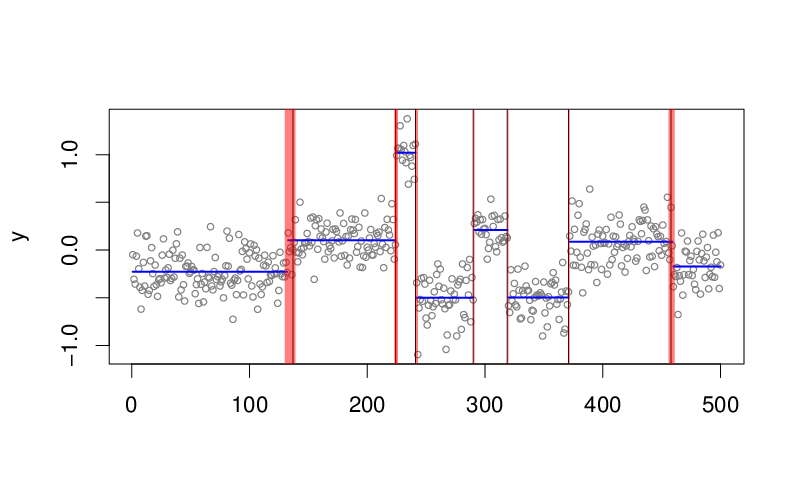## Convergence issue¶

We set up a simple simulated example with two change points very close together.

In :
set.seed(1)
x = rnorm(100)
x[50:51]=x[50:51]+8
x.s = susie_trendfilter(x,0,L=2,estimate_prior_variance=TRUE)
plot(x,col=gray(0.5),ylab="y",xlab="",cex=0.65)
lines(predict(x.s),type="s",lwd=2)
dev.off()

png: 2
In :
%preview cp_susie_convergence_bad.pdf -s png --dpi 100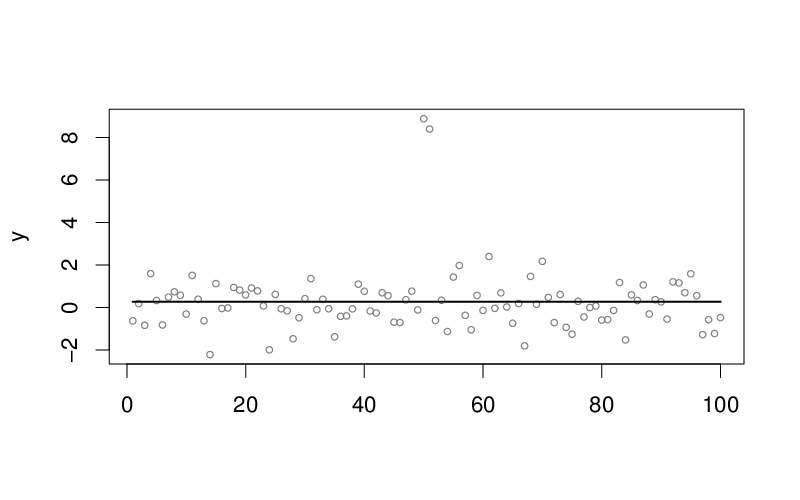In :
susie_get_objective(x.s)

-181.835041124445

Now initialize at the "truth",

In :
s0 = susie_init_coef(c(49,51),coef_value = c(8,-8),p=100)
x.s2 = susie_trendfilter(x,0,s_init=s0,estimate_prior_variance=TRUE)
pdf("cp_susie_convergence_okay.pdf", width=8, height=5, pointsize=16)
plot(x,col=gray(0.5),ylab="y",xlab="", cex=0.65)
lines(predict(x.s2),col=2,type="s", lwd=2)
dev.off()

png: 2
In :
%preview cp_susie_convergence_okay.pdf -s png --dpi 100

%preview cp_susie_convergence_okay.pdf
> cp_susie_convergence_okay.pdf (5.7 KiB):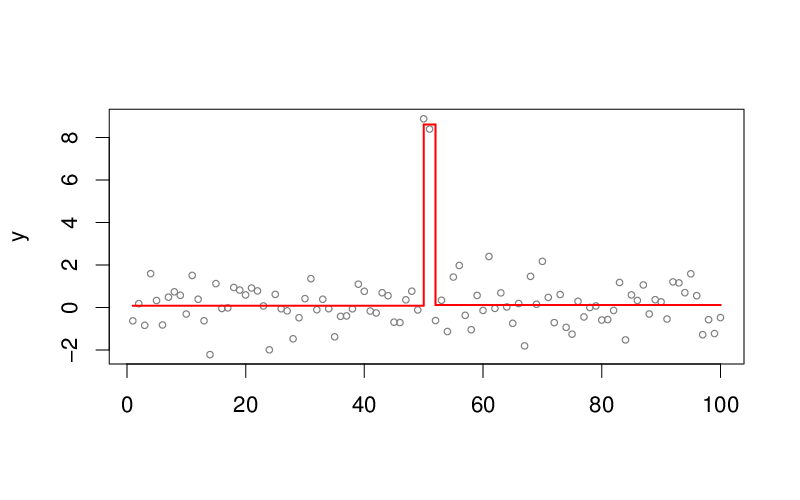The objective is better compared to previous fit,

In :
susie_get_objective(x.s2)

-148.217576587086

## Figure for the manuscript¶

Putting these plots together,

In :
pdf("cp_susie.pdf", width=8, height=6, pointsize=16)
par(mfrow=c(2,1),mar=c(2,4,2,2), cex.axis=0.8)

plot(genomdat,col=gray(0.5), ylab="y", xlab="variable", cex=0.65)
segments(x0=test2$output$loc.start,x1=test2$output$loc.end,y0=test2$output$seg.mean,col="blue",lwd=2)
abline(v=cumsum(c(137,87,17,49,29,52,87))) # changepoint locations
plot_cs(genomdat.s)

plot(x,col=gray(0.5), ylab="y", xlab="variable", cex = 0.65)
lines(predict(x.s),type="s", lwd=2)
lines(predict(x.s2),col=2, type="s", lwd=2)
dev.off()

png: 2
In :
%preview cp_susie.pdf -s png --dpi 100

%preview cp_susie.pdf
> cp_susie.pdf (10.6 KiB):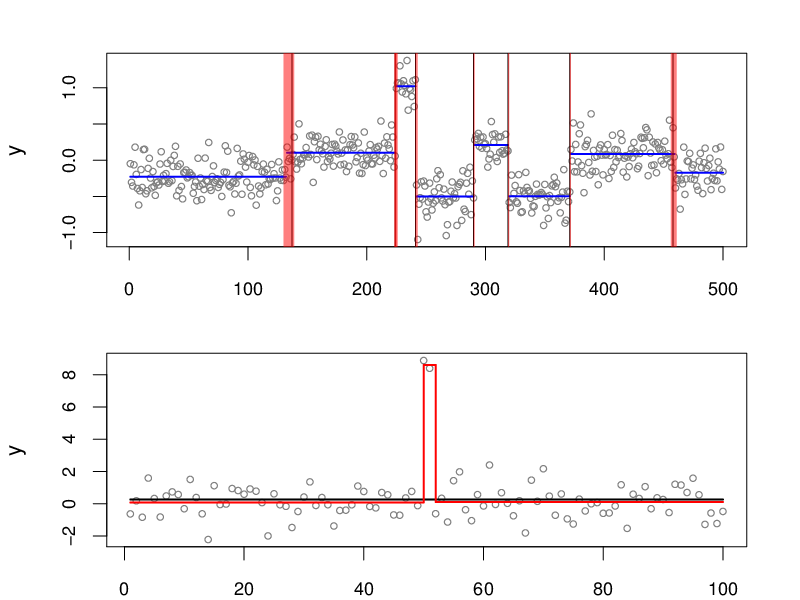In :
%sessioninfo


SoS

SoS Version 0.17.5

R

Kernel ir R R version 3.5.1 (2018-07-02) Platform: x86_64-pc-linux-gnu (64-bit) Running under: Scientific Linux 7.4 (Nitrogen) Matrix products: default BLAS/LAPACK: /software/openblas-0.2.19-el7-x86_64/lib/libopenblas_haswellp-r0.2.19.so locale:  LC_CTYPE=en_US.UTF-8 LC_NUMERIC=C  LC_TIME=en_US.UTF-8 LC_COLLATE=en_US.UTF-8  LC_MONETARY=en_US.UTF-8 LC_MESSAGES=en_US.UTF-8  LC_PAPER=en_US.UTF-8 LC_NAME=C  LC_ADDRESS=C LC_TELEPHONE=C  LC_MEASUREMENT=en_US.UTF-8 LC_IDENTIFICATION=C attached base packages:  stats graphics grDevices utils datasets methods base other attached packages:  changepoint_2.2.2 zoo_1.8-4 DNAcopy_1.56.0 susieR_0.6.2.0405 loaded via a namespace (and not attached):  Rcpp_0.12.19 magrittr_1.5 getPass_0.2-2  uuid_0.1-2 lattice_0.20-38 stringr_1.3.1  tools_3.5.1 grid_3.5.1 htmltools_0.3.6  matrixStats_0.54.0 digest_0.6.17 crayon_1.3.4  Matrix_1.2-15 IRdisplay_0.5.0 repr_0.15.0  base64enc_0.1-3 IRkernel_0.8.12.9000 evaluate_0.11  pbdZMQ_0.3-3 stringi_1.2.4 compiler_3.5.1  expm_0.999-3 jsonlite_1.5 

© 2017-2018 authored by Gao Wang at Stephens Lab, The University of Chicago

Exported from manuscript_results/changepoint_example.ipynb committed by Gao Wang on Wed Dec 19 00:30:12 2018 revision 3, fe1be96